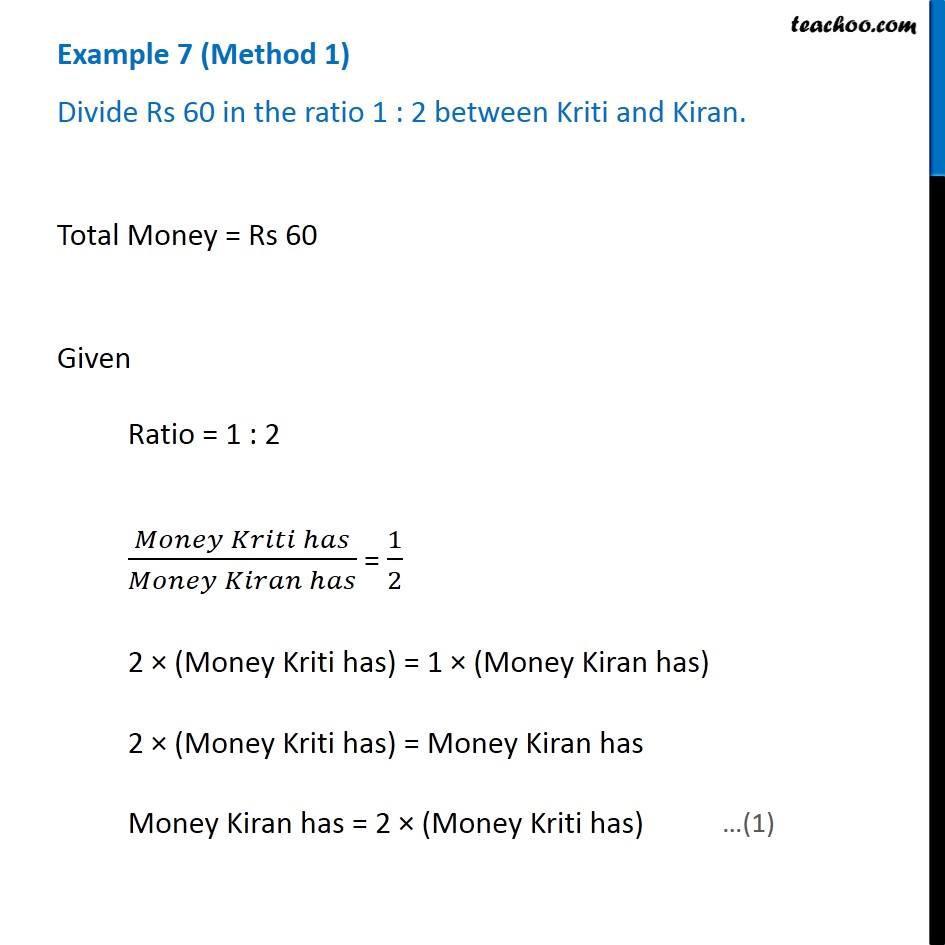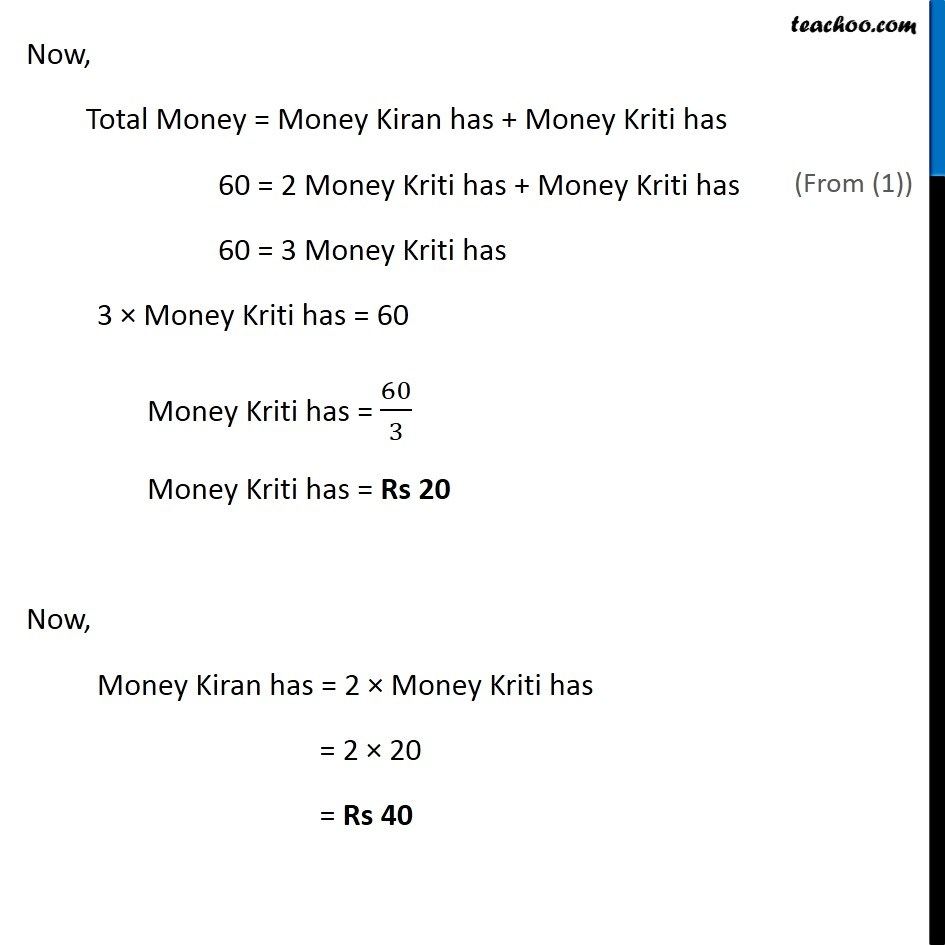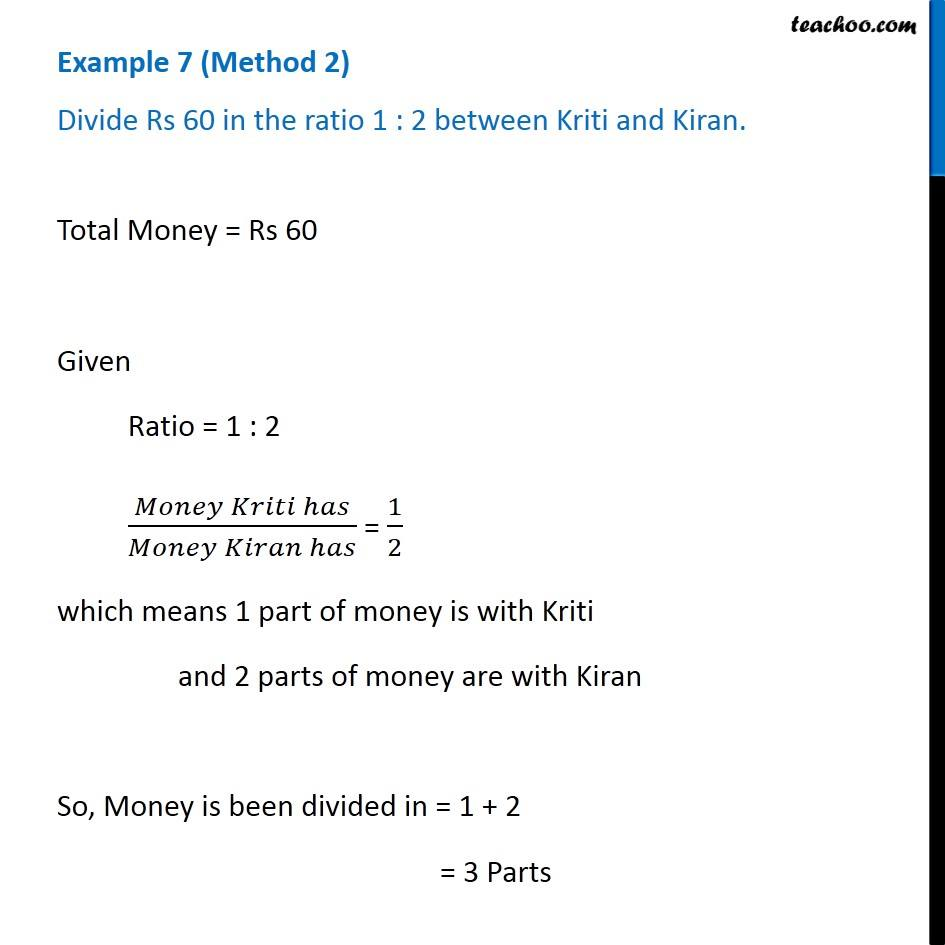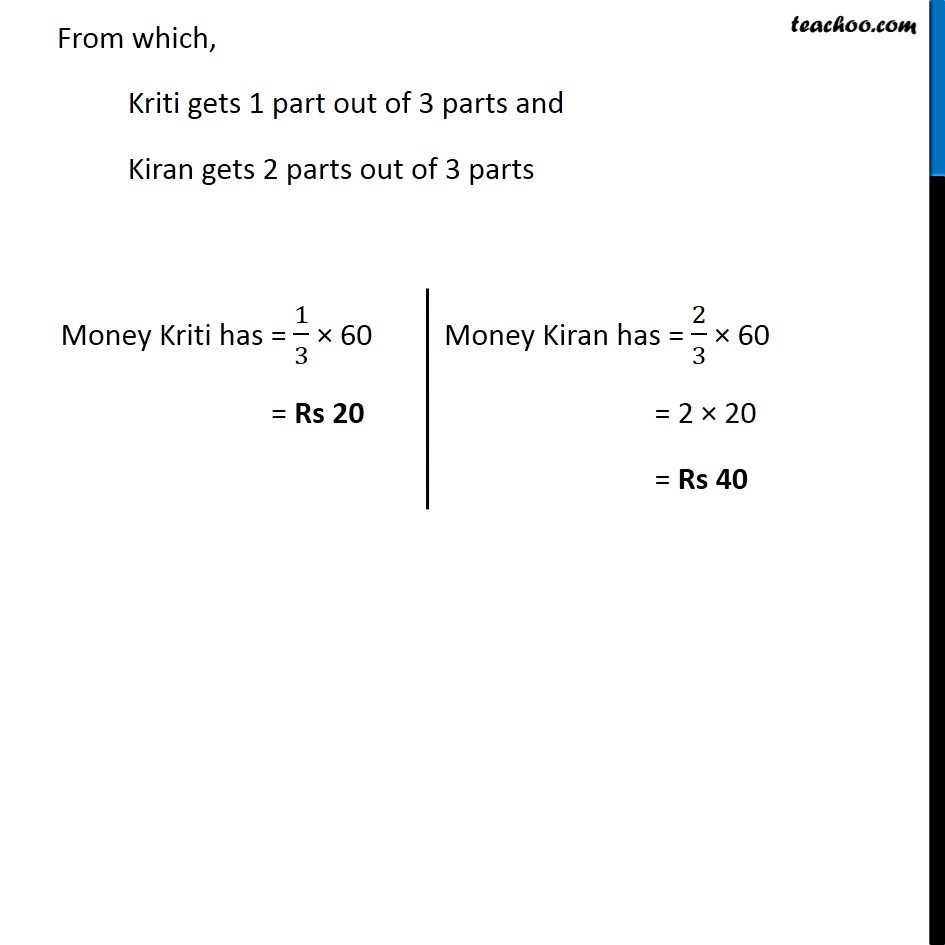Examples

Chapter 12 Class 6 Ratio and Proportion
Serial order wiseLearn in your speed, with individual attention - Teachoo Maths 1-on-1 Class

### Transcript

Example 7 (Method 1) Divide Rs 60 in the ratio 1 : 2 between Kriti and Kiran. Total Money = Rs 60 Given Ratio = 1 : 2 (𝑀𝑜𝑛𝑒𝑦 𝐾𝑟𝑖𝑡𝑖 ℎ𝑎𝑠)/(𝑀𝑜𝑛𝑒𝑦 𝐾𝑖𝑟𝑎𝑛 ℎ𝑎𝑠) = 1/2 2 × (Money Kriti has) = 1 × (Money Kiran has) 2 × (Money Kriti has) = Money Kiran has Money Kiran has = 2 × (Money Kriti has) Now, Total Money = Money Kiran has + Money Kriti has 60 = 2 Money Kriti has + Money Kriti has 60 = 3 Money Kriti has 3 × Money Kriti has = 60 Money Kriti has = 60/3 Money Kriti has = Rs 20 Now, Money Kiran has = 2 × Money Kriti has = 2 × 20 = Rs 40 Example 7 (Method 2) Divide Rs 60 in the ratio 1 : 2 between Kriti and Kiran.Total Money = Rs 60 Given Ratio = 1 : 2 (𝑀𝑜𝑛𝑒𝑦 𝐾𝑟𝑖𝑡𝑖 ℎ𝑎𝑠)/(𝑀𝑜𝑛𝑒𝑦 𝐾𝑖𝑟𝑎𝑛 ℎ𝑎𝑠) = 1/2 which means 1 part of money is with Kriti and 2 parts of money are with Kiran So, Money is been divided in = 1 + 2 = 3 Parts From which, Kriti gets 1 part out of 3 parts and Kiran gets 2 parts out of 3 parts Money Kriti has = 1/3 × 60 = Rs 20 Money Kiran has = 2/3 × 60 = 2 × 20 = Rs 40